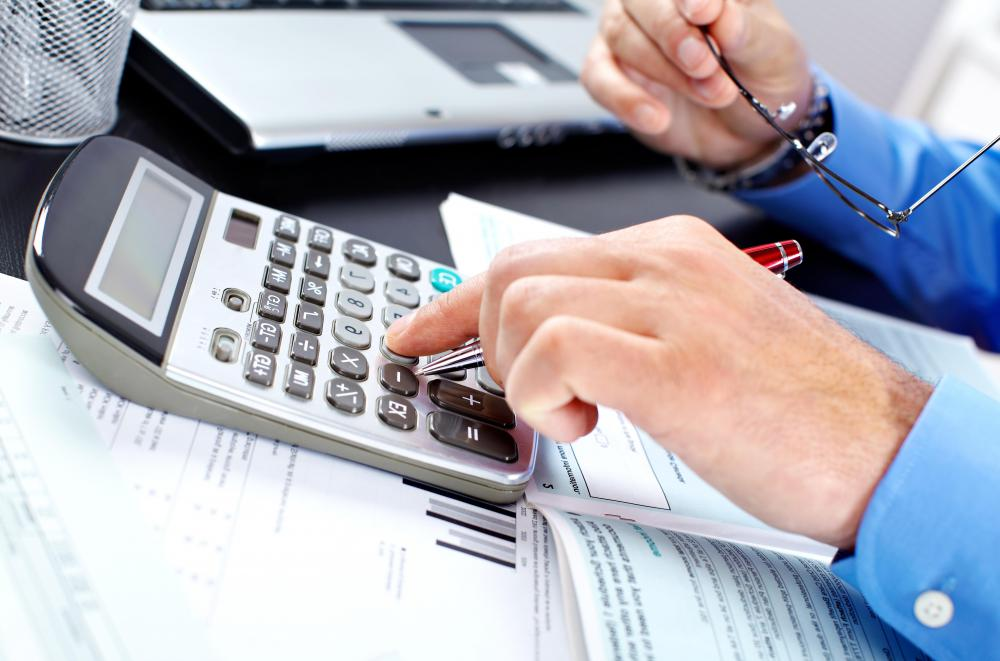# How do I Calculate Accumulated Depreciation?

Jim B.

Accumulated depreciation refers to the amount of value that has been lost by a business asset over the time that it has been used. This concept is separate from the depreciation expense, which shows up on an income statement and does not add up from year to year. To calculate the accumulated depreciation of a business asset, the depreciation expenses from all of its years in use are totaled. The amount of depreciation each year depends on the method of depreciation that is used on a particular asset.Business assets lose value over time, and calculating accumulated depreciation helps determine when to invest in new assets.

Any asset, whether it's a car, computer, or a piece of machinery, that is used by a business for more than a year and is used for business purposes is said to depreciate in value each year. This means that it loses value from its original cost, usually due to the wear and tear it receives during its life. Businesses are allowed to write off the loss in value on their tax returns. As an asset continues to be used, the total amount of value lost due to that wear and tear is known as accumulated depreciation.

To calculate accumulated depreciation, simply add up the yearly depreciation amounts for the asset up to that point. For example, imagine an asset that has been in use for three years by a business. The yearly depreciation amounts up to that point were \$500 US Dollars (USD) for the first year, #300 USD for the second year, and \$200 for the third year. In that case, the accumulated depreciation for that asset up to that point is \$500 USD plus \$300 USD plus \$200 USD, which equals \$1,000 USD.

It's important to note that this concept is separate from the depreciation expense. Depreciation expense is usually found on a company's yearly income statement, as opposed to accumulated depreciation, which is normally found on a company's balance sheet. Another difference is that the depreciation expense is a one-year amount and does not add up from year to year.

There are different methods used to calculate depreciation, and whatever method is used will have an effect on the total amount of depreciation that an asset undergoes. The simplest method of depreciation, known as the straight-line method, allows for assets to be depreciated at the same amount every year. In some cases, businesses may wish to take the biggest financial hit on an asset in the year in which it is purchased. For such cases, the declining balance method of depreciation, in which a fixed percentage rate is applied to the balance of the cost of the asset, may be used.

## You might also Like